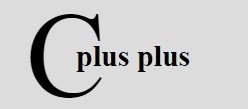# C++ Function Types

In C++, there are broadly two types of functions :

1. Built-in (library) functions
2. User-defined functions

## C++ Built-in Functions

These functions are part of the compiler package. These are part of standard library made available by the compiler. For example, exit(), sqrt(), pow(), strlen() etc. are library functions (built-in functions). Built-in functions are already discussed in C++ Standard Library Functions Tutorial.

Built-in functions helps you, to use function without declaring and defining it. To use built-in functions in a program, you have to only include that header file where the required function is defined. Here is an example. This program uses built-in function named strlen() of string.h header file to find the length of the string.

```/* C++ Function Types - C++ Built-in Function */

#include<iostream.h>
#include<string.h>
#include<conio.h>
void main()
{
clrscr();
char str;
cout<<"Enter any string (line):\n";
cin.getline(str, 80);
int len = strlen(str);
cout<<"\nLength of the string is: "<<len;
getch();
}```

Here is the sample run of the above C++ program, finds the length of the string entered by the user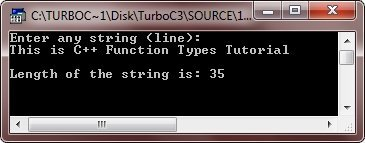## C++ User-defined Functions

The user-defined functions are created by you i.e., the programmer. These functions are created as per requirements of your program. Here is an example, demonstrating user-defined functions in C++

```/* C++ Function Types - C++ User-defined Function */

#include<iostream.h>
#include<conio.h>
void start_msg(void);    // function declaration
void main()
{
clrscr();
start_msg();     // function calling
int num;
cout<<"Enter a number: ";
cin>>num;
cout<<"You entered: "<<num;
getch();
}

void start_msg(void)   // function definition
{
cout<<"Welcome to codescracker.com\n";
cout<<"This is C++ Function Types Tutorial\n\n";
}```

Here is the sample output of the above C++ program: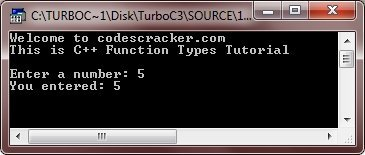Here is another program in C++, also demonstrating user-defined function

```/* C++ Function Types - C++ User-defined Function */

#include<iostream.h>
#include<conio.h>
void greeting(void);
void main()
{
clrscr();
char ch;
cout<<"Press y for greeting: \n";
cin>>ch;
if(ch=='y' || ch=='Y')
{
greeting();
}
cout<<"Want to learn more ? (y/n).. ";
cin>>ch;
if(ch=='y' || ch=='Y')
{
cout<<"Yep..!!..You can proceed..!!\n";
}
else
{
cout<<"Sure..!!..You can take rest..!!\n";
}
getch();
}

void greeting(void)
{
cout<<"Welcome to codescracker.com";
cout<<"\nYou are at good position now\n\n";
}```

Here are some sample runs of the above C++ program: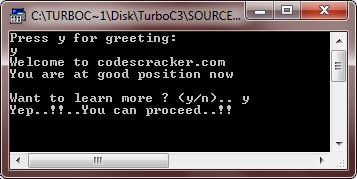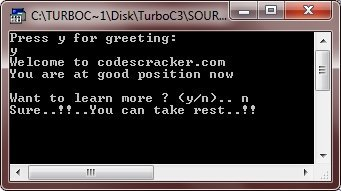Let's take another program, for complete understanding of user-defined functions in C++

```/* C++ Function Types - C++ User-defined Function */

#include<iostream.h>
#include<conio.h>
void msg(void);
int add(int, int);
void main()
{
clrscr();
msg();
int a, b;
cout<<"Enter any two number: ";
cin>>a>>b;
cout<<"The sum of the two numbers is: "<<add(a, b);
getch();
}

void msg(void)
{
cout<<"Welcome to codescracker.com";
cout<<"\nThis is addition program\n\n";
}

int add(int x, int y)
{
return x+y;
}```

Below is the sample output of this C++ program:Tools
Calculator

Quick Links
Signup - Login - Give Online Test